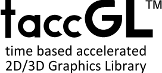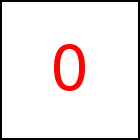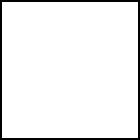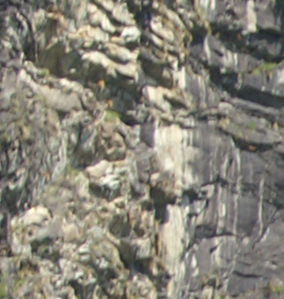# Some First Examples - taccGL™ Canvas Library Tutorial

## First Example

A taccgl™-animation is a collection of taccgl™-transitions. Each transition shows one or more visual effect(s) (e.g. a motion, a color, or blend effect) of a single actor. Actors can be very different things, ranging from simple geomentical shapes like triangles and rectangles over (images of) HTML elements, possibly mapped on boxes, cylinders etc. to 3D-objects created with a 3D modelling program.

taccgl™-animations are initially set up by javaScript. Then they run independently (if possible on the GPU), in parallel to the normal operation of the web page (until altered or controlled by further javasSript programs). For example the following javaScript code

 var transition = taccgl.actor('firstdiv'); transition . rotateMiddle(1,0,0);transition . duration(5);transition . start(); RUN

starts rotating this paragraph, i.e. the HTML element with the id="firstdiv". The var statement in the first line defines a single transition named transition. Then transition. rotateMiddle(1, 0, 0) defines this transition to perform, when executing, a rotation around around the (1,0,0) axis (i.e.the x axis). transition. duration(5) selects a 5 seconds duration for the transition. Finally transition.start() starts the transition. Please click on the -button above to run the example.

Although the example at a first glance might look as a simple CSS animation, taccgl™ in fact bypasses the DOM, CSS, and javaScript bottelneck of browsers and almost completely performes the animations via WebGL™ inside the GPU. Therefore animations are very fast (provided there is good GPU support) and because no DOM nodes are created, animations can have huge numbers of actors. Try yourself to run the example or or slice/split the element into , , or slices and rotate these individually in a sequence.

### Concise Notation

The example can be rewritten in the following more concise notion (using taccgl™s Fluent Interface; the transition methods rotateMiddle and duration return the current transition object as result):

 taccgl.actor('firstdiv') . rotateMiddle(1,0,0) . duration(5). start(); RUN

This notion can also be read as follows: first select an element using actor, then set transition kind(s) and parameters. Later we will explain, that instead of just one element multiple elements can be selected. It is also possible to select other items, such as parts or slices of elements, 3D objects from model files, etc. and then set transition kind(s) and parameters for all the items at once.

### Running the Example

To run the first example on your pages you need to include the taccgl™ library in the head or your document and somewhere place a link to start the animation Show full sample code.

## Second Example : External 3D Model

The second example animates a 3D model created with a 3D modelling program such as Blender. The actor method generally has a second parameter, that determines the shape or kind of the actor to be shown. The first parameter denotes an HTML element which defines where the actor should appear.

<div id="examplediv"
style="width:150px;
height:150px;
border:1px solid black">

Placeholder example DIV

</div>

The first line of this example loads the 3D model. The second line is very similar to the first example above. It just adds a second parameter to the actor method loadedModel.scene() to show the 3D model. rotatemiddle, duration, and start are used as in the first example.

In this example the designated HTML element ("examplediv" in this case) acts as a placeholder, where the 3D object is shown. The element itself is hidden while the animation is playing. In contrast in the following example, the HTML element is mapped on the 3D object:

Multiple 3D Labels
can be placedYou can place various External 3D Models on a single HTML page and align them with different HTML elements, and combine them with various shapes Basic Shapes. It is also possible to select objects from an external 3D model and to apply various animations, .

See the full sample code of the second example. This also demonstrates the use of a javaScript function for writing the animation, which is very helpful for animations longer than the first example.

## Third Example(s) : Combination of Multiple Motions and Transitions

<div id="examplediv2"
style="width:150px;
height:150px;
border:1px solid black">

Placeholder example DIV

</div>

The following examples show three ways of combining the rotation from the first example with a linear motion. Using from({x:0,oy:1000}) a motion from x-coordinate 0 and from 1000px below is specified. The first example creates two transitions and shows two copied of the blue box, one copy rotating and one copy moving. The second example applies both motions to the same transition showing the blue box rotating and moving simultaneously. The third example creates two transitions that via the cont() functions perform sequentially, first move and then rotate the blue box.

 taccgl.actor('examplediv2') . rotateMiddle(0,0,1) . start(); taccgl.actor('examplediv2') . from({x:0,oy:1000}) . start(); RUN taccgl.actor('examplediv2') . rotateMiddle(0,1,0) . from({x:0,oy:1000}) . start(); RUN taccgl.actor('examplediv2') . from({x:0,oy:1000}) . start(). cont() . rotateMiddle(0,1,0) . start(); RUN

## Fourth Examples : Box and FlexibleIn the next example the HTML element with id Rock2 is an image showing the texture from a rock. You can map this HTML element on a 3D-Box and move and rotate this box. actor gets the second parameter taccgl.dddBox in this case. taccgl™ can display various Basic Shapes this way.

One of these is called taccgl.flexiBorder. Is deforms the surface of the HTML element according to a mathematical function: In the second example this is a Circle and in the third example a cylinder.

 taccgl.actor('Rock',taccgl.dddBox) . rotateMiddle(1,1,1). from({ox:-500,oy:-500,oz:1400}) . duration(5). start(); RUN taccgl.actor('Rock',taccgl.flexiBorder) . Circle1 () . duration(5). start(); RUN taccgl.actor('Rock',taccgl.flexiBorder) . nparts(50) .Flip(0,0,6*Math.PI/4,6*Math.PI/4) .rotateMiddle (1,1,0). duration(5). start(); RUN

## Fifth Examples : Multiple Selectors

It is possible to define transitions on multiple elements with a single statement, such as rotating all links on this page. taccgl.mTagName('a') selects all HTML elements with tag name a (i.e. all a tags). The second example below makes all h2-headlines move in from the right and slow down smoothly (Accelerated Motion, vEnd).

 taccgl.mTagName('a') . paint(). hide(). visAtEnd() . rotateMiddle(0,1,0) . start(); RUN taccgl.mTagName('h2') . paint(). hide(). visAtEnd() . from({x:0,rx:-1}).vEnd(0,0,0) . start(); RUN

Also parts of HTML elements can be selected. sliceH(5) for example cuts the selected Rock element into 5 horizontal slices. sequence (4) then performs the specified animation for each part in a sequence and showAfter() shows the animated parts also after the motion is finished.

 taccgl.ma('Rock2'). paint(). sliceH(5). to({oy:-500}) . sequence (4). showAfter(). start() RUN taccgl.ma('Rock2'). paint(). sliceH(20). to({oy:-500}) . sequence (4). showAfter(). start() RUNUsing sliceH and sliceV an element can be cut into horizontal parts and then each into vertical pieces.

The mp method gets a function as parameter that is performed for each element individually. In the second example below mp( t=> t.startTime(Math.random())) is used to assign each part an random starting time.

The third and fourth examples are a bit more complicated and described in detail in section Selectors for Multiple Transitions.

 taccgl.ma('Rock2'). paint(). hide(). visFinal() . sliceH(3). sliceV(3).rotateMiddle(.7,.7,0) . sequence(5,-3). showBefore(). showAfter(). start(); RUN taccgl.ma('Rock2') . paint(). hide(). visFinal() . sliceH(3). sliceV(3).rotateMiddle(.7,.7,1) . mp( t=> t.startTime(Math.random())). showBefore(). showAfter(). start(); RUN taccgl.ma('Rock2'). paint() . hide(). visFinal() . showbefore(). sliceH(24) . sliceV(24) . alpha(1,-0.3). dur(2) .mp ( t=>{t . to({ox:2000*Math.random()-1000,oy:2000*Math.random()-1000}) .posZ(0,-4000) .vEnd(0,0,0)} ) . start(); RUN taccgl.ma('thisbox'). paint() . hide() . visFinal() . showbefore() . sliceH(24) . sliceV(24) . alpha(1,‑0.3). dur(2) .mp ( t=>{t . to({ox:2000*Math.random()‑1000,oy:2000*Math.random()‑1000}) . posZ(0,‑4000) .vEnd(0,0,0)}) . start(); RUN

WebGL™ is a trademark of the Khronos Group Inc.

Next Page:Basic Shapes
Previous Page: Tutorial

# Tutorial

taccgl™ - HTML5 Canvas Library